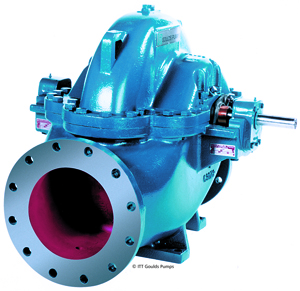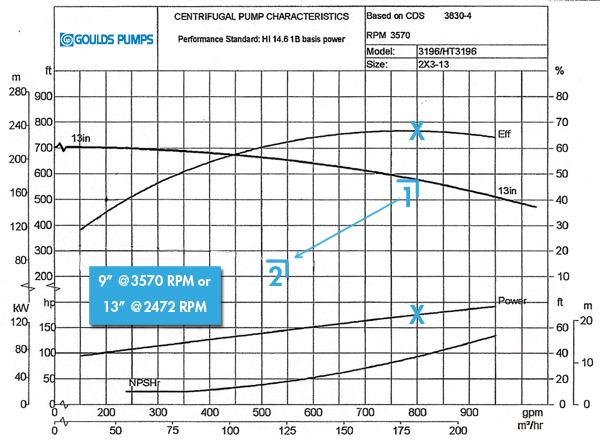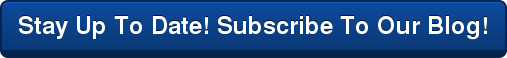####920-733-4425# How To Use Affinity Laws to Calculate A New Best Efficiency PointWhen selecting a new pump, an engineer will review pump curves to make sure the pump chosen will run at the best efficiency point for the given application. But what if one of the variables in the application needs to change, and the curve that came perfectly packaged with the pump no longer applies? If no curve exists, should the pump be replaced with another that has the curve you need? Of course not. Using the affinity laws, it is possible to determine approximate curves to estimate the centrifugal pump's new best efficiency point.

Centrifugal pump affinity laws provide a method to determine a new pump operating point when a performance curve that indicates performance with different impeller diameters or operating speeds is not available.

Now that we understand what centrifugal pump affinity laws are, let's take a look at the equations that will help you determine your new pump operating point.

With the speed held constant, the following relationships apply:

• Flow, typically expressed in gallons per minute (GPM) is proportional to velocity or impeller diameter
• Head, typically expressed in feet of water (FT), is proportional to the square of the velocity or impeller diameter
• Power, typically expressed in horsepower (HP), is proportional to the cube of the velocity or impeller diameter which follows since horsepower is calculated by multiplying flow times head

This relationship is expressed mathematically as follows where D = impeller diameter:With the impeller diameter held constant, the following relationships apply:

• Flow is proportional to velocity or pump speed, typically expressed in revolutions per minute (RPM)
• Head is proportional to the square of the velocity or pump speed
• Power is proportional to the cube of the velocity or pump speed

This relationship is expressed mathematically as follows:Let's put these equations into an example. Hypothetically, let's say we have a pump with the initial operating point (1):

• Flow (Q) = 800 GPM
• Horsepower (P) = 175 HP
• Impeller diameter = 13"
• Pump speed = 3570 RPM

The desire is to determine what the pump output would be using a 9" impeller and what the corresponding reduced speed would be to provide the same pump output with a 13" impeller. Using the affinity laws, the following results were obtained:### Mike Baxter

Mike Baxter is a Professional Engineer, and the National Accounts Manager for Crane Engineering. He has over 35 years of experience in chemical, pulp and paper, food and beverage, power generation, OEM, as well as water and wastewater industries. His focus is on assisting customers with fluid process needs, including pumping, filtration, mixing, and packaged skid systems.